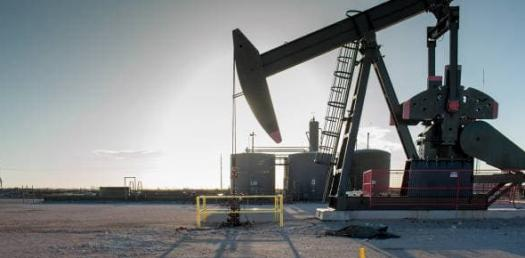# Gas's And Equlibrium Quiz

9 Questions | Total Attempts: 164SettingsChemistry multiple choice questions

• 1.
Boyles Law deals with what?
• A.

Pressure and volume

• B.

Volume and temperature

• C.

Temperature and pressure

• D.

Both a and b

• E.

Both a and c

• 2.
What is the ideal gas law?
• A.

P1v1/t1=p2v2/t2

• B.

PH=-log(H+)

• C.

PV=NRT

• D.

D=m/v

• E.

P=trw

• 3.
Which way would the equibrium shift for the equation, if you increase the temperature?
• A.

Shift left

• B.

Shift right

• C.

Nothing happens

• D.

Shift up

• E.

Shift down

• 4.
Taking away heat favors what reaction?
• A.

Endothermic

• B.

Chemical

• C.

Covalent

• D.

Exothermic

• E.

None of the above

• 5.
Gay-Lussac’s law deal with?
• A.

Pressure& volume

• B.

Volume& temperature

• C.

Temperature & pressure

• D.

Both a and b

• E.

None of the above

• 6.
How many torr. are there if there is 3.5 atm
• A.

2945

• B.

2740

• C.

2720

• D.

760

• E.

1400

• 7.
What is the volume of any gas at STP?
• A.

1 mole

• B.

1atm

• C.

760 torr.

• D.

• E.

22.4L

• 8.
If there is .75 atm of H2, How many mmHG are there?
• A.

730MMhg

• B.

512MMhg

• C.

570MMhg

• D.

112MMhg

• E.

750MMhg

• 9.
Charles’s law deal with?
• A.

Temperature & pressure

• B.

Volume& temperature

• C.

Pressure& volume

• D.

All of the above

• E.

None of the above

Related TopicsBack to top